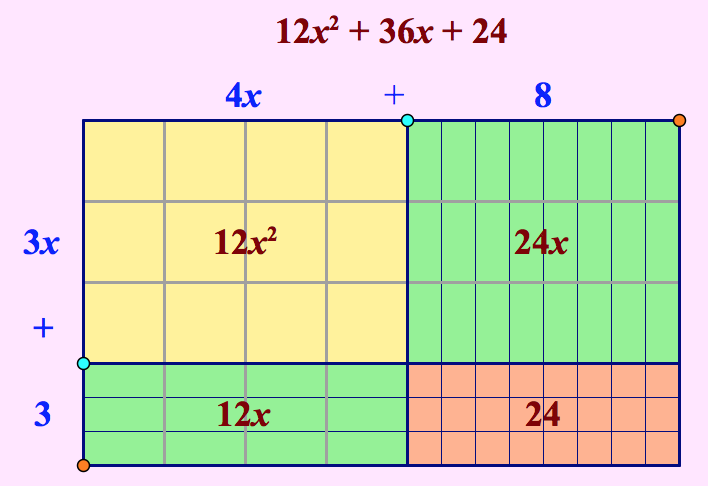# Binomial Multiplication and Factoring Games

The four Web Sketchpad activities below from our Dynamic Number project  provide a sequenced collection of challenges and games that develop an area model approach to binomial multiplication and factoring. You can click any of the images to open the interactive websketches on a separate page.

## Dynamic Algebra Tiles, Part One

In the first websketch, students use a grid of virtual algebra tiles to interpret and solve binomial multiplication problems. They focus on problems of the form (ax + b)(cx + d), where the values of a, b, c, and d are positive integers. Students begin by noting the areas of the three types of tiles: yellow (x2), green (x), and orange (1). They then determine the area of the yellow region, the two green regions, and the orange region. They relate the area of the grid to the dimensions of its sides (3x + 3 and 4x + 8 in the example below), and add the partial products to determine the area of the grid and the product of the binomials.  To create new problems, students drag the blue and orange points.## Dynamic Algebra Tiles, Part Two

In the second websketch, students again use a grid of virtual algebra tiles to interpret and solve binomial multiplication problems. They focus on problems of the form (ax + b)(cx + d), where b and/or d is  negative Our teacher notes for this activity explain how to discuss the meaning of the negative values in the context of algebra tiles.## Factoring Games, Part One

In the next websketch,  students play games in which they factor quadratic expressions using virtual algebra tiles. They play each game multiple times, with random challenges generated by Web Sketchpad, and work to improve their score. In the problem below, students must factor 6x2 + 19x + 15 using the grid. By dragging the blue and orange points, students create the two factors, 3x + 5 and 2x + 3.

If students are certain of their answer, they can press Next Problem. They will be taken to the next random problem to solve and receive full credit (initially 10 points) for a correct answer and 0 points for an incorrect answer. If students are uncertain of their answer, they can press Check Answer. Doing so gives them the opportunity to retry the problem if they solved it incorrectly, but the value of a correct problem will be reduced by 2 points with each answer check. Students can also press Show Hints if they need help solving a problem. This reveals the partial products, but students will lose 2 points of the remaining number of available points.## Factoring Games, Part Two

In the final websketch, students play a game in which they find the binomial factors of quadratic expressions. Unlike the previous game, this game does not represent each problem with virtual algebra tiles. Rather, the randomly-generated problems are presented with a minimal of visual imagery to prepare students for a more abstract understanding of factoring. Drag the blue and green sliders to adjust the factors.## One thought on “Binomial Multiplication and Factoring Games”

1.Katie says:

This is great! I would love to see something similar for distributing a monomial through brackets (binomial or trinomial)